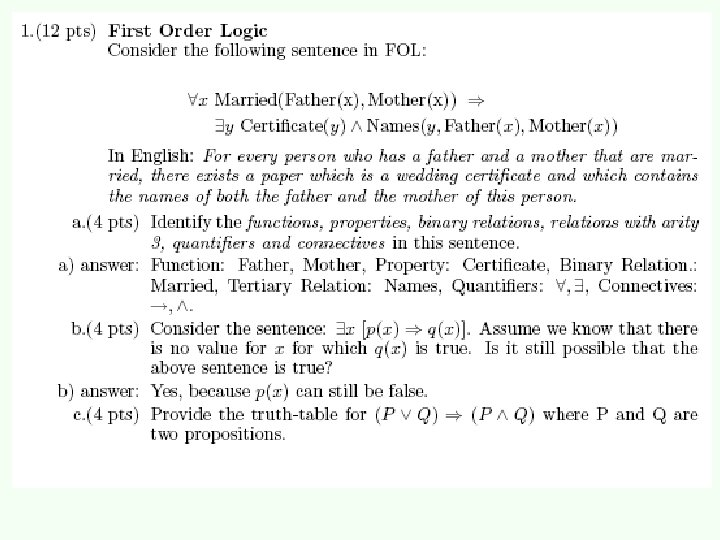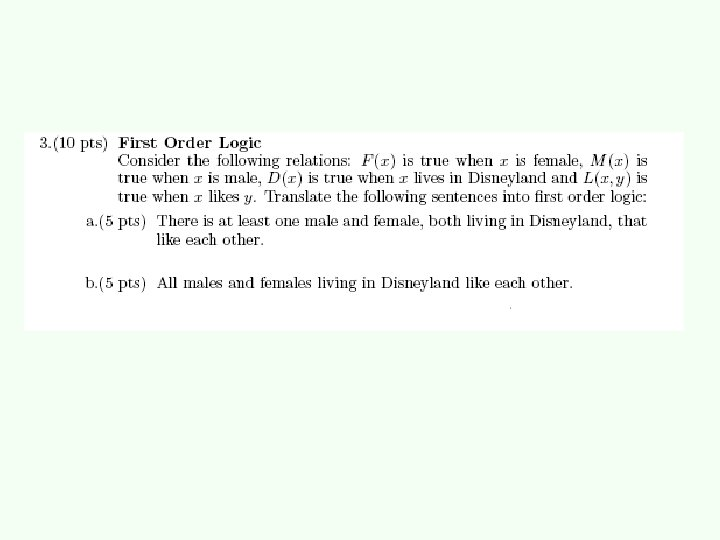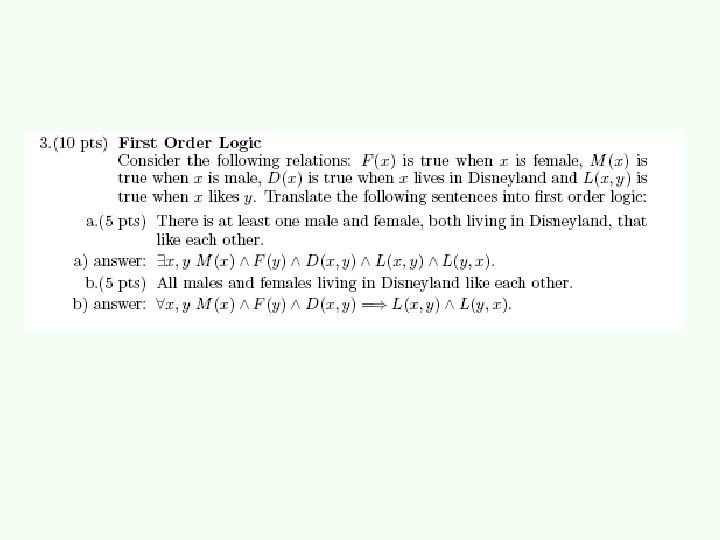# FirstOrder Logic Chapter 8 Problem of Propositional Logic

• Slides: 22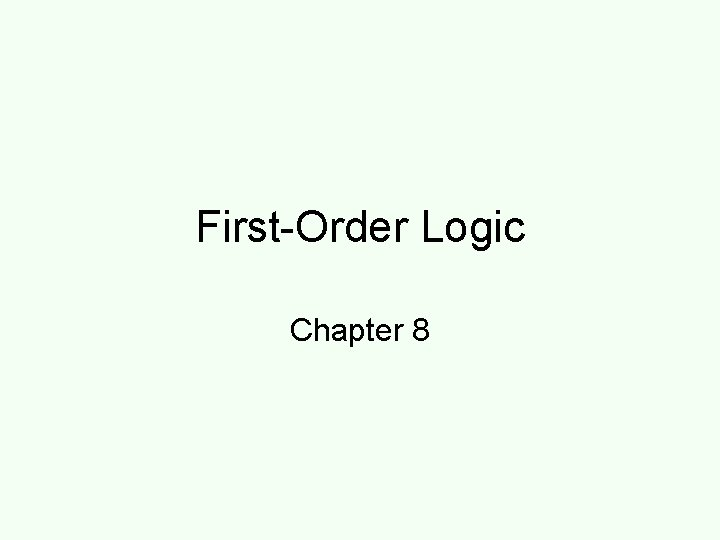First-Order Logic Chapter 8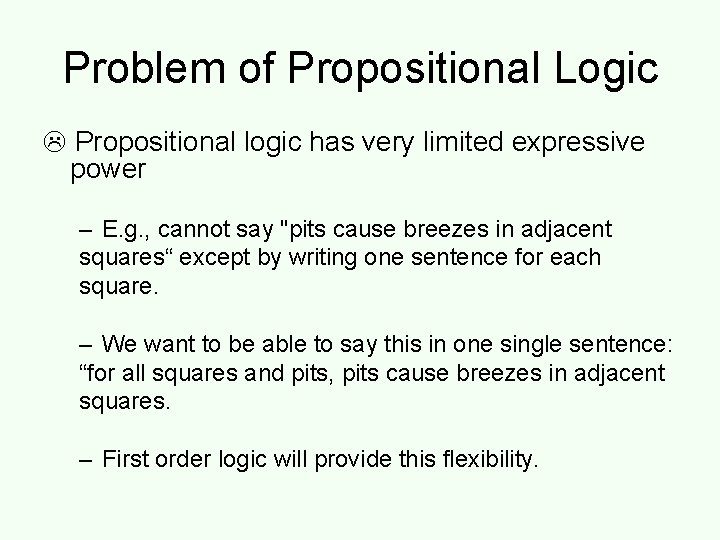Problem of Propositional Logic Propositional logic has very limited expressive power – E. g. , cannot say "pits cause breezes in adjacent squares“ except by writing one sentence for each square. – We want to be able to say this in one single sentence: “for all squares and pits, pits cause breezes in adjacent squares. – First order logic will provide this flexibility.First-order logic • Propositional logic assumes the world contains facts that are true or false. • First-order logic assumes the world contains – Objects: people, houses, numbers, colors, baseball games, wars, … – Relations between objects: red, round, prime, brother of, bigger than, part of, comes between, …Relations • Some relations are properties: they state some fact about a single object: Round(ball), Prime(7). • n-ary relations state facts about two or more objects: Married(John, Mary), Largerthan(3, 2). • Some relations are functions: their value is another object: Plus(2, 3), Father(Dan).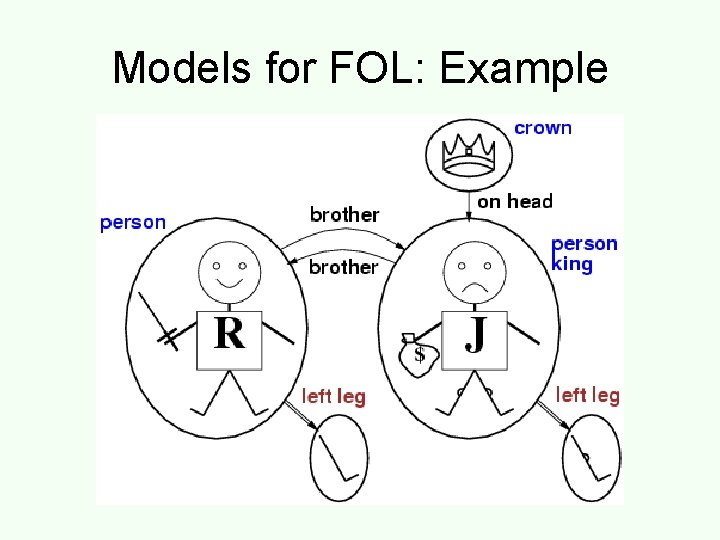Models for FOL: ExampleAtomic Sentences • Sentences in logic state facts that are true or false. • Properties and n-ary relations do just that: Larger. Than(2, 3) (means 2>3) is false. Brother(Mary, Pete) is false. • Note: Functions do not state facts and form no sentence: Brother(Pete) refers to the object John (his brother) and is neither true nor false. • Brother(Pete, Brother(Pete)) is True. Binary relation FunctionComplex Sentences • We make complex sentences with connectives (just like in proposition logic). binary relation property function objects connectivesQuantification • Round(ball) is true or false because we give it a single argument (ball). • We can be much more flexible if we allow variables which can take on values in a domain. e. g. reals x, all persons P, etc. • To construct logical sentences we need a quantifier to make it true or false.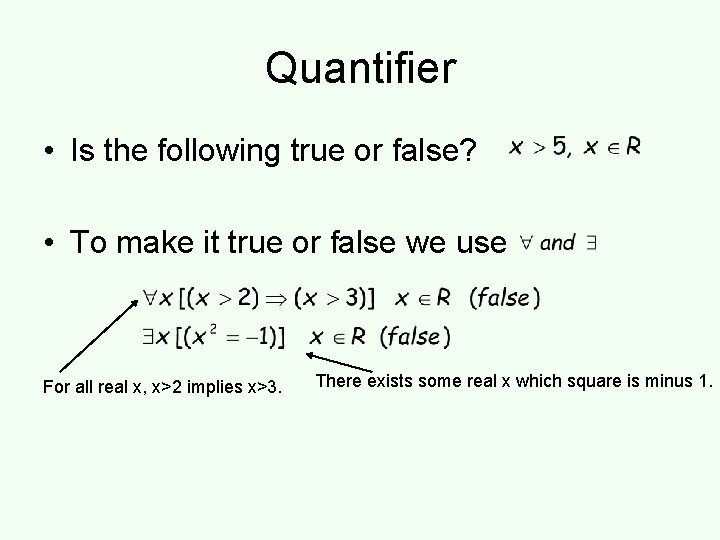Quantifier • Is the following true or false? • To make it true or false we use For all real x, x>2 implies x>3. There exists some real x which square is minus 1.Nested Quantifiers • Combinations of universal and existential quantification are possible: Binary relation: “x is a father of y”. Quiz : which is which: Everyone is the father of someone. Everyone has everyone as a father There is a person who has everyone as a father. There is a person who has a father There is a person who is the father of everyone. Everyone has a father.De Morgan’s Law for Quantifiers De Morgan’s Rule Generalized De Morgan’s Rule is simple: if you bring a negation inside a disjunction or a conjunction, always switch between them (or and, and or). • Equality symbol: Father(John)=Henry. This relates two objects.Common mistakes to avoid • is the main connective with • is the main connective with All of these must be true! King(Pete) AND Person(Pete) King(Mary) AND Person(Mary) King(Tablespoon) AND Person(Tablespoon) False! too strong One of these should be true! if King(Pete) then Person(Pete) if King(Mary) then Person(Mary) If King(Tablespoon) then Person(Tablespoon) True! too weak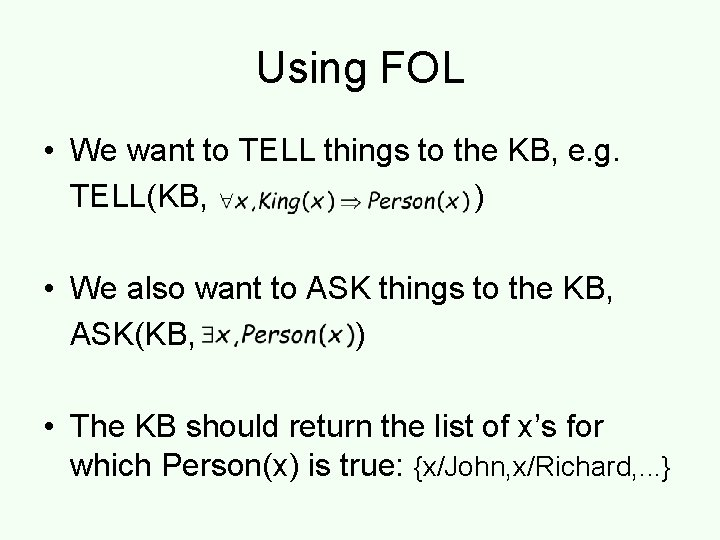Using FOL • We want to TELL things to the KB, e. g. TELL(KB, ) • We also want to ASK things to the KB, ASK(KB, ) • The KB should return the list of x’s for which Person(x) is true: {x/John, x/Richard, . . . }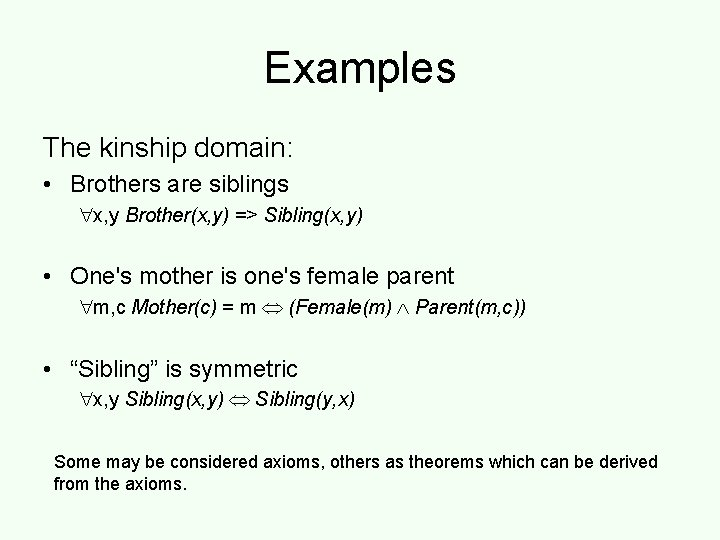Examples The kinship domain: • Brothers are siblings x, y Brother(x, y) => Sibling(x, y) • One's mother is one's female parent m, c Mother(c) = m (Female(m) Parent(m, c)) • “Sibling” is symmetric x, y Sibling(x, y) Sibling(y, x) Some may be considered axioms, others as theorems which can be derived from the axioms.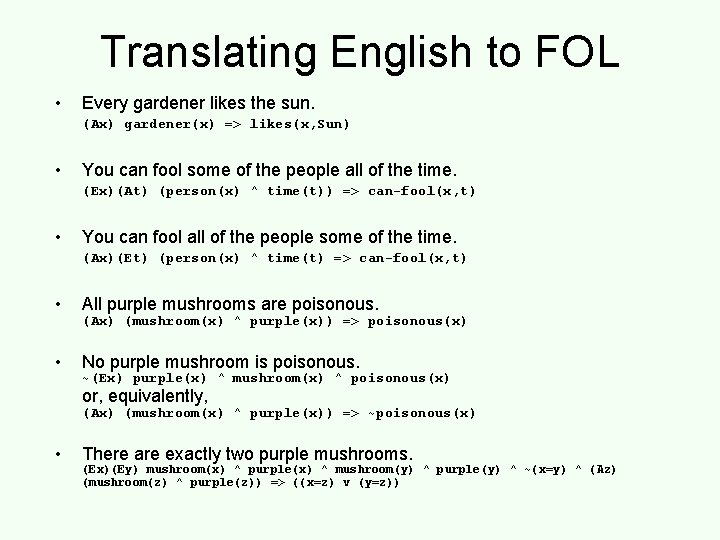Translating English to FOL • Every gardener likes the sun. (Ax) gardener(x) => likes(x, Sun) • You can fool some of the people all of the time. (Ex)(At) (person(x) ^ time(t)) => can-fool(x, t) • You can fool all of the people some of the time. (Ax)(Et) (person(x) ^ time(t) => can-fool(x, t) • All purple mushrooms are poisonous. • No purple mushroom is poisonous. (Ax) (mushroom(x) ^ purple(x)) => poisonous(x) ~(Ex) purple(x) ^ mushroom(x) ^ poisonous(x) or, equivalently, (Ax) (mushroom(x) ^ purple(x)) => ~poisonous(x) • There are exactly two purple mushrooms. (Ex)(Ey) mushroom(x) ^ purple(x) ^ mushroom(y) ^ purple(y) ^ ~(x=y) ^ (Az) (mushroom(z) ^ purple(z)) => ((x=z) v (y=z))